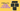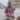# 4 Ways to Swap Variables in JavaScript

Posted February 19, 2020Start discussion

A lot of algorithms require swapping 2 variables.

During a coding interview, you could be asked “How to swap 2 variables without a temporary variable?”.

It’s good to know multiple ways to perform swapping of variables. In this post, you will read about 4 ways to swap (2 that use additional memory and 2 that don’t).

## 1. Destructuring assignment

Destructuring assignment (a feature of ES2015) lets you extract items of an array into variables. For example, the following code destructures an array:

``````let a;
let b;

[a, b] = [1, 2, 3];
a; // => 1
b; // => 2``````

`[a, b] = [1, 2, 3]` is a destructuring assignment that destructures `[1, 2, 3]` array. `a` variable is assigned with the first item `1` of `[1, 2, 3]`, correspondingly `b` is assigned with the second item `2`.

Knowing how to destructure an array, it’s easy to use it for swapping variables. Let’s swap the variables `a` and `b` using destructuring assignment:

``````let a = 1;
let b = 2;

[a, b] = [b, a];
a; // => 2
b; // => 1``````

`[a, b] = [b, a]` is the destructuring assignment that swaps the variables `a` and `b`.

At the first step, on the right side of the destructuring, a temporary array `[b, a]` (which evaluates to `[2, 1]`) is created.

Then the destructuring of the temporary array occurs: `[a, b] = [2, 1]`. The variable `a` is assigned with `2`, and `b` with `1`. The swapping of `a` and `b` has been performed.

I like the destructuring approach because it’s short and expressive: swapping is performed in just one statement. It works with any data type: numbers, strings, booleans, objects.

I recommend swapping variables using a destructuring assignment for most of the cases.

## 2. Temporary variable

Swapping variables using a temporary variable is classic. As the name suggests, this approach requires an additional temporary variable.

Let’s swap the values of variables `a` and `b` using a temporary variable `temp`:

``````let a = 1;
let b = 2;
let temp;

temp = a;a = b;b = temp;
a; // => 2
b; // => 1``````

`temp` is the temporary variable.

At the first step, `temp` is assigned with the value of `a`. Then `a` variable is assigned with the value of `b`. Finally, the variable `b` is assigned with the value of `temp` (having the initial value of `a`).

The swapping of variables using a temporary variable works with any value type, like numbers, strings, booleans, objects.

The downside of this approach is the need for a specialized temporary variable, plus the swapping happens in 3 statements.

You can swap variables without the use of additional memory (like a temporary array or variable).

The following example swaps the variables `a` and `b` using the addition `+` and difference `-` arithmetic operators:

``````let a = 1;
let b = 2;

a = a + b;b = a - b;a = a - b;
a; // => 2
b; // => 1``````

Initially, `a` is `1` and `b` is `2`. Let’s see how the 3 statements perform the swapping:

1. `a = a + b` assigns to `a` the value `1 + 2`.
2. `b = a - b` assigns to `b` the value `1 + 2 - 2 = 1` (`b` is now `1`).
3. `a = a - b` assigns to `a` the value `1 + 2 - 1 = 2` (`a` is now `2`).

Finally, `a` is `2` and `b` is `1`. The swapping of `a` and `b` has been performed.

While this approach doesn’t use temporary variables, it has considerable limitations.

First, you can swap integers only. Secondly, be aware of the numbers overflow when performing the addition at the first step `a = a + b` (the sum must be lower than `Number.MAX_SAFE_INTEGER`).

## 4. Bitwise XOR operator

The XOR operator evaluates to true if the operands are different. As a reminder, here’s the XOR truth table:

``````┌─────┬─────┬───────┐
│ a   │ b   │ a ^ b │
├─────┼─────┼───────┤
│ 0   │ 0   │   0   │
│ 1   │ 1   │   0   │
│ 0   │ 1   │   1   │
│ 1   │ 0   │   1   │
└─────┴─────┴───────┘``````

In JavaScript, the bitwise XOR operator `n1 ^ n2` performs the XOR operation on each bit of `n1` and `n2` numbers.

For example, here’s how `5 ^ 7` evaluates to `2`:

``````1 0 1 (5 in binary)
1 1 1 (7 in binary)
-----
0 1 0 (5 ^ 7 = 2 in binary)``````

Bitwise XOR has 2 interesting properties:

• `n ^ n = 0`: bitwise XOR performed on the same number is `0`
• `n ^ 0 = n`: bitwise XOR performed on a number and zero is the same number

These XOR properties can be used to swap variables. Let’s see how to swap `a` and `b` variables:

``````let a = 1;
let b = 2;

a = a ^ b;b = a ^ b;a = a ^ b;
a; // => 2
b; // => 1``````

Here’s an explanation why the swapping works:

1. `a = a ^ b`
2. `b = a ^ b`. Based on 1 `a` is substituted with `a ^ b`. Thus `b = (a ^ b) ^ b = a ^ (b ^ b) = a ^ 0 = a`. Remember than `b` is now `a`.
3. `a = a ^ b`. Based on 1 `a` is substituted with `a ^ b` and based on 2 `b` is substituted with `a`. Thus `a = (a ^ b) ^ a = b ^ (a ^ a) = b ^ 0 = b`. The variable `a` becomes `b`.

If you find the explanation complicated, feel free to skip it. The properties of bitwise XOR (`n ^ n = 0` and `n ^ 0 = n`) composed in 3 assignments lets you swap the values of `a` and `b`.

Swapping variables using bitwise XOR operator has limitations: you can swap only integers.

## 5. Conclusion

JavaScript offers a bunch of good ways to swap variables, with and without additional memory.

The first way, which I recommend for daily use, is swapping variables by applying destructuring assignment `[a, b] = [b, a]`. It’s a short and expressive approach.

The second way uses a temporary variable. It’s a good alternative to the destructuring assignment approach.

The third way, using addition and subtraction, doesn’t use additional variables or memory. However, the approach is limited to swapping integer numbers only.

In the same way, the fourth approach using bitwise XOR doesn’t use additional memory. But again, you can swap integers only.

What’s your preferred way to swap variables?

## Quality posts into your inbox

I regularly publish posts containing:

• Important JavaScript concepts explained in simple words
• Overview of new JavaScript features
• How to use TypeScript and typing
• Software design and good coding practices